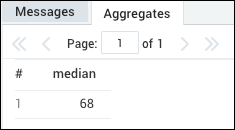# median Grouping Operator

In order to calculate the median value for a particular field, you can utilize the `pct` (percentile) operator with a percentile argument of 50.

## Syntax​

``pct(<field> [, 50]) [as <field>] [by <field>]``

## Rules​

• Creates a field with the naming convention: `_<field>_pct_50`

## Examples​

For example:

``* | parse "data=*" as data  | pct(data, 50) as median``

To calculate the median value of a field called `"Len: *"` as seconds, and then take the median, use the following query:

``| parse "Len: *" as seconds| pct(seconds,50) as median``

Which would return results similar to:Legal
Privacy Statement